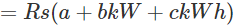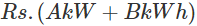# Cost of Electrical Energy

There are three kinds of expenditures involved in generating electricity. These are fixed cost, semi-fixed cost, running or operating cost.

## Fixed Cost of Electricity

In every manufacturing unit there is some hidden expenditure which fixed. This is same for manufacturing one unit or thousand units of the items. In electric generating station like manufacturing unit, there are some hidden costs which independent of the quantity of electricity produced. These fixed expenditures are mainly due to an annual cost to run the organization, interest on capital cost and tax or rent of the land on which the organization established, salaries of high officials and interests of loans (if any) on the capital cost of the organization. Like these main costs, there are many others expenditures which do not change whether the rate of production of electrical energy units is less or more.

## Semi-fixed Cost of Electricity

There is another type costing for any manufacturing or production or any similar type of industries. These costs are not strictly fixed and also not fully dependent on the number of items manufactured or produced. These costs depend on the size of the plant. These actually depend on the assumption of a maximum number of items which can be produced from the plant at a time during peak demand period. That means the forecasted production demand of the plant determines how big will be the manufacturing or production plant. Likewise, the size of an electrical generating plant depends on the maximum demand of the connected load of the system. If the maximum demand of the load is quite higher than the average demand of the load, then the power generating plant should be constructed and well equipped to fulfill that maximum demand of the system even the peak demand lasts for less than an hour. This type of costs is referred as semi-fixed cost. It is directly proportional to the maximum demand on the power station. The annual interest and depreciation on capital investment of building and equipment, taxes, salaries of management and clerical staff, expenditure for installation etc. come under semi-fixed costs.

## Running Cost of Electricity

The concept of running cost is quite simple. It solely depends on the number of units produced or generated. In power generating plant the main running cost is the cost of fuel burnt per unit of electrical energy generation. The cost of lubricating oil, maintenance, repairs and salaries of operating staff are also accounted under running cost of the plant. Since these charges are directly proportional to the number of units generated. For generating more units of electrical energy required running expenditures are more and vice versa.
Hope you got the basic concept of the cost of electrical energy.

## Expressions for Cost of Electrical Energy

The total cost of per unit generation of electrical energy can be expressed in the following ways.
First, we have to calculate the entire expenditure of the plant including the organization which is fixed throughout the year and accounted for a fixed cost. Say this is a. This is considered as fixed cost for entire electrical energy generated in the year.
In the same way, we have to calculate the total semi-fixed cost of the plant throughout the year. The semi-fixed cost is proportional to maximum demand of the plant. So, we have to find the maximum demand of the year. So the proportionality constant b can easily be calculated. Therefore, the semi-fixed cost of the plant for the year is b(maximum demand kilowatt).
Now, we will calculate entire running expenses of the plant for producing total kWh units of energy generated in the year. If c is the running cost per unit of generated electricity then 0
Total cost of the plant for producing entire electric throughout the year isSometimes it is assumed that entire capital cost and other costs except for the running expenses for producing electricity entirely depend on the maximum demand of the plant. In that case, it is assumed that there is no absolute fixed cost. The expression for the annual cost of energy then becomesWhere A is the cost per unit /maximum demand and B is the running cost of producing one unit of electrical cost.

Want To Learn Faster? 🎓
Get electrical articles delivered to your inbox every week.
No credit card required—it’s 100% free.